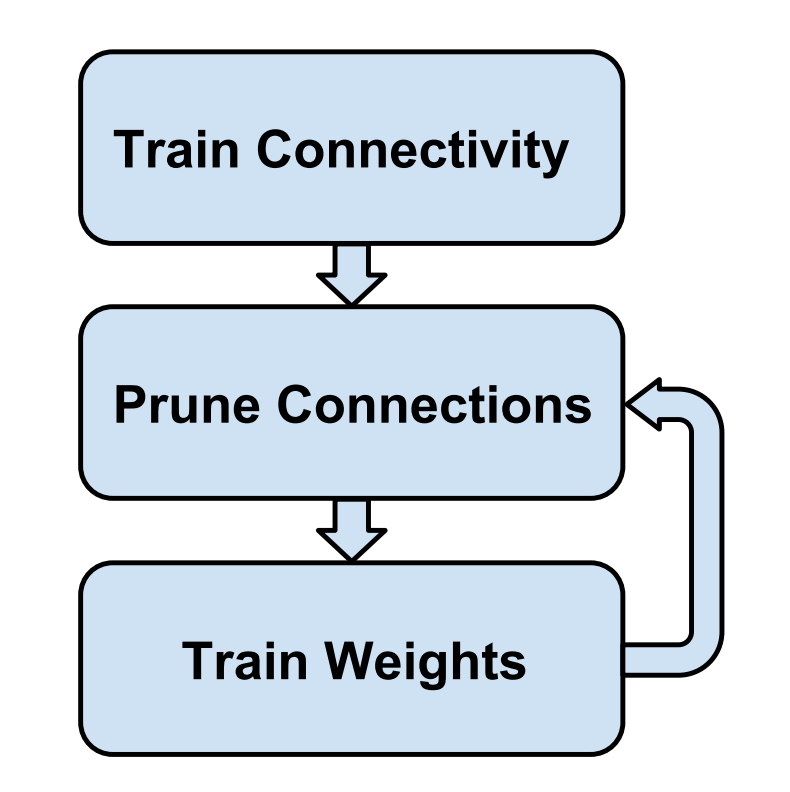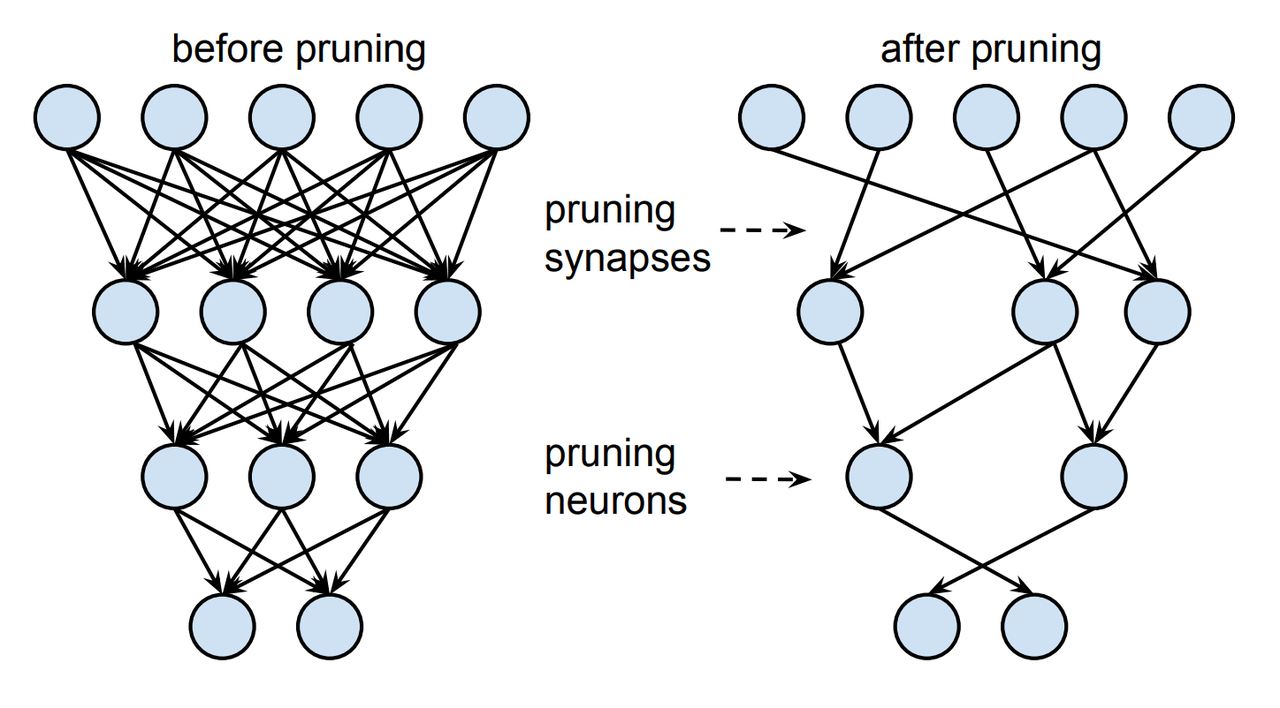# NN models compression techniques

Recent research of the neural networks mainly focused on the improving accuracy. Despite this, there are exist a lot of methods to reduce models size and speed up them. Here I will try briefly discuss each of methods and compare them among each other.

## Pruning

Pruning initially was proposed on Jun 2015 at Learning both Weights and Connections for Efficient Neural Networks paper. Main idea of this method is:

• Train whole network
• Prune some connections that less than required threshold
• Retrain pruned network. If we not perform retraining model accuracy can be decreased a lot.

Last two steps can be repeated several times.Training pipeline, image source

As the result we will receive such representation of the model:Pruned model, image source

During pruning such additional updates should be done to the model:

• The pruning threshold is chosen as a quality parameter multiplied by the standard deviation of a layer’s weights.
• Use L2 regularization to push the weights near to zero. In paper was reported that use of the L1 regularization is unreasonable.
• Update dropout is some exist with such rule. It can updated as $$D_{r} = D_{o} \sqrt{\frac{C_{ir}}{C_{io}}}$$, where:
• $$D_{r}$$ - our new dropout rate
• $$D_{o}$$ - previous dropout rate
• $$C_{io}$$ - number of connections in layer $$i$$ in original network
• $$C_{ir}$$ - number of connections in layer $$i$$ in the network after retraining
• Learn model after pruning with lower learning rate.
• Convert model to sparse matrix.
• Batch norm?? Hm, I think it didn’t exist yet when pruning was proposed
• Authors said that we can 2x reduce number of the neurons even without retraining

For training in some framework:

• Train network as usual
• For pruning we can just multiply each layer with binary mask
• Perform retraining with layer initialized from previous network
• Convert retrained network to sparse tensors